# The Cosine Function in Right Triangles

Cosine is a trigonometric ratio comparing two sides of a right triangle. Cosine is usually shortened to cos but is pronounced cosine. This function can be used to determine the length of a side of a triangle when given at least one side of the triangle and one of the acute angles.

Quick Review: the three main trig ratios are sine, cosine and tangent. They can be memorized using SOH CAH TAH What does this mean? It means that cosine is the ratio of the adjacent side divided by the hypotenuse.

Cosθ =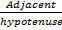Let's look at an example at how cosine can be used to find the length of the opposite side.To find x write an equation using the cosine ratio and then solve for x

cos 30° =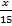multiply both sides of the equation by 15

(15) cos 30 =(15)     You will need to use a calculator to find the value of cos 30°

(15) (.8660) = xmake sure your calculator is in degree mode by verifying that cos 30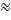.8660 (rounded to 4 decimal places)

12.99 = x The adjacent side has an approximate length of 12.99 or 13 if rounded to the nearest tenth.

Now let's look at how Cosine can be used to find the length of the hypotenuse.To find x write an equation using the cosine ratio and then solve for x

Cos 20° =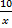Multiply both sides of the equation by x.

(x) cos 20° =(x)             You will need to use a calculator to find the value of cos 20°. Round to 4 decimal places

Make sure your calculator is in degree mode by verifying that (x) (.9397) = 10     cos 20.9397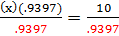Divide both sides by .9397 to isolate x

x = 10.6    the length of the hypotenuse is approximately 10.6

The trig ratios have many real world and practical applications in fields such as aviation, architecture, surveying. Using the trigonometric ratios, such as cosine, allows for the measurement of things that cannot be determined using typical measurement tools.

 Related Links: Math Trigonometry The Sine Function in Right Triangles The Tangent Function in Right Triangles

To link to this The Cosine Function in Right Triangles page, copy the following code to your site: